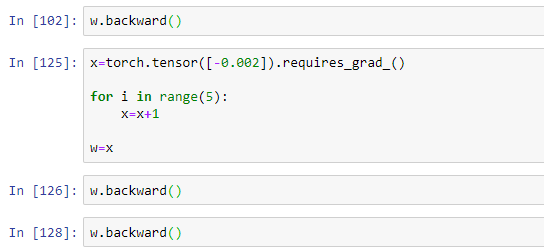# Why autograd graph is not freed?

Why the autograd graph is not freed after w.backward()?
It should have been freed, no retrain_graph=True.

The original `w` tensor is replaced by the `x+i` operation, which doesn’t need to store any intermediate activations and thus allows you to call `w.backward()` multiple times.

Thank you for your reply. But does that mean no looping network is created if replacing can be done?

There won’t be a “looping network”, since you are not creating a sequence of operations, but are rerunning `x + i`.
A graph would be created e.g. if you would assign the result back to `x`.

``````x=torch.tensor(10.).requires_grad_()

z=torch.empty(1)
for i in range(5):
w=x*2+i

w.backward() #works
w.backward() # grads freed, does not work

``````

why this does not work?

``````x=torch.tensor(10.).requires_grad_()

z=torch.empty(1)
for i in range(5):
w=(x-1)-i

w.backward() #works
w.backward() # works
``````

Why the above works?

``````x=torch.tensor(10.).requires_grad_()

z=torch.empty(1)
for i in range(5):
w=(x/1)-i

w.backward() #works
w.backward() # grads freed, does not work
``````

Why above one does not work?

Does pytorch treat +/- differently than * or / because of how derivatives are calculated for them? As for +/- upward gradient is just distributed, but for * and / there are actually different ways to calculate derivative?

So, pytorch create graphs to determine gradients, simple as that. If network only distributes gradient, then there are no intermediate operations to be freed?w.backward() is working but if `x=x*1 +1` then `w.backward()` can not be called after calling it earlier. Why?

I think intermediate activations, when created and when removed is the reason.

Yes, the key difference is that +/- doesn’t need any saved tensors (just shapes for broadcasting) while * and / do need the inputs.
This is also a reason why you can inplace-modify sometimes and sometimes hit the “a value needed for backward has been inplace modified” (or whatever, from the top of my head) error.

Best regards

Thomas